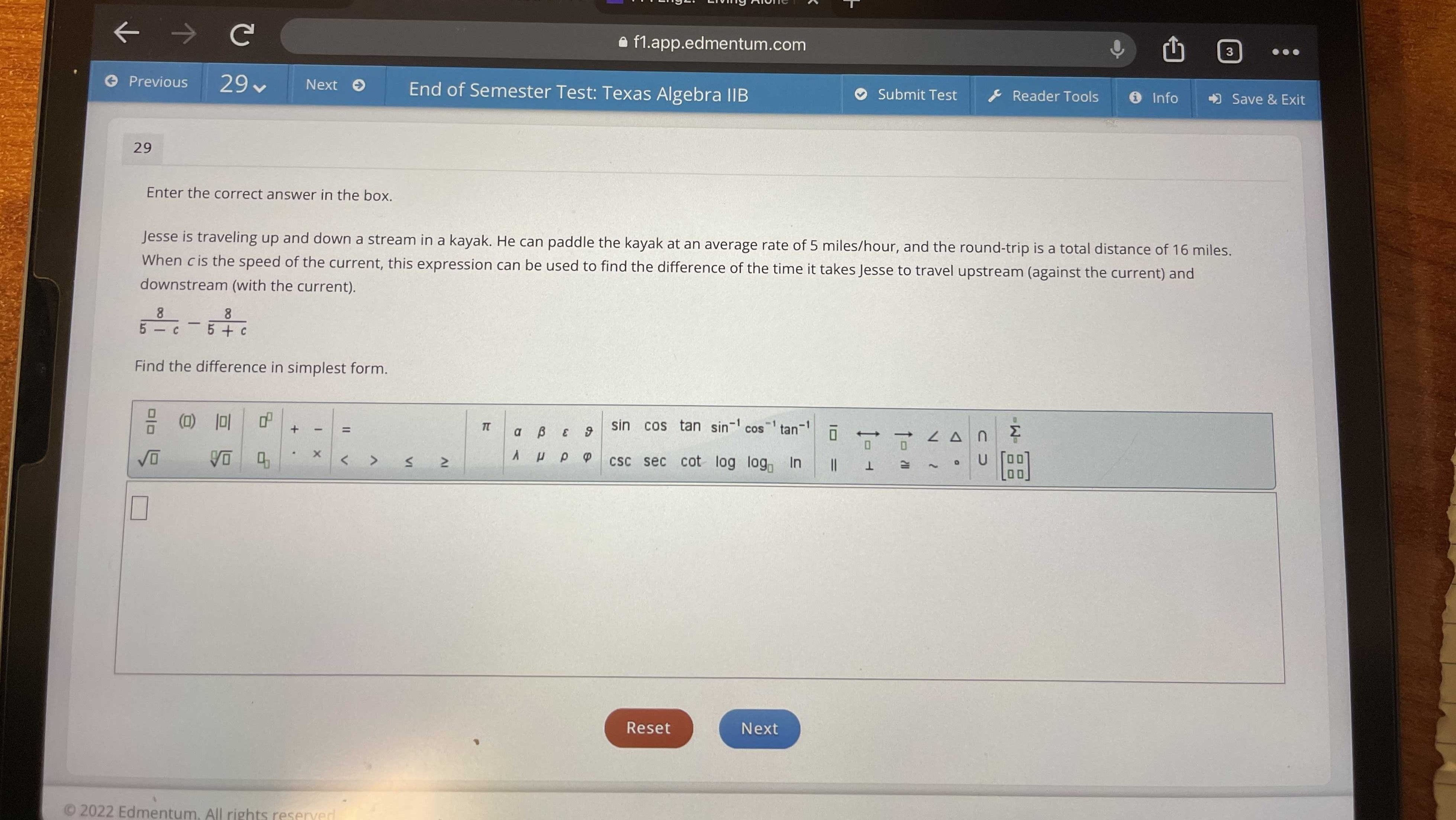### Still have math questions?Jesse is traveling up and down a stream in a kayak. He can paddle the kayak at n average rate of 5 mile/hour, and the round-trip is a total distance of 16 miles.When c is the speed of the current,this expression can be used to find the difference of the time it takes Jesse to travel upstream (against the current) and downstream (with the current). $$\frac { 8} { 5- x} - \frac { 8} { 5+ x}$$
$$\frac { 40+ 8c- 40+ 8c} { 25- c^ { 2} }$$=$$\frac { 16c} { 25- c^ { 2} }$$